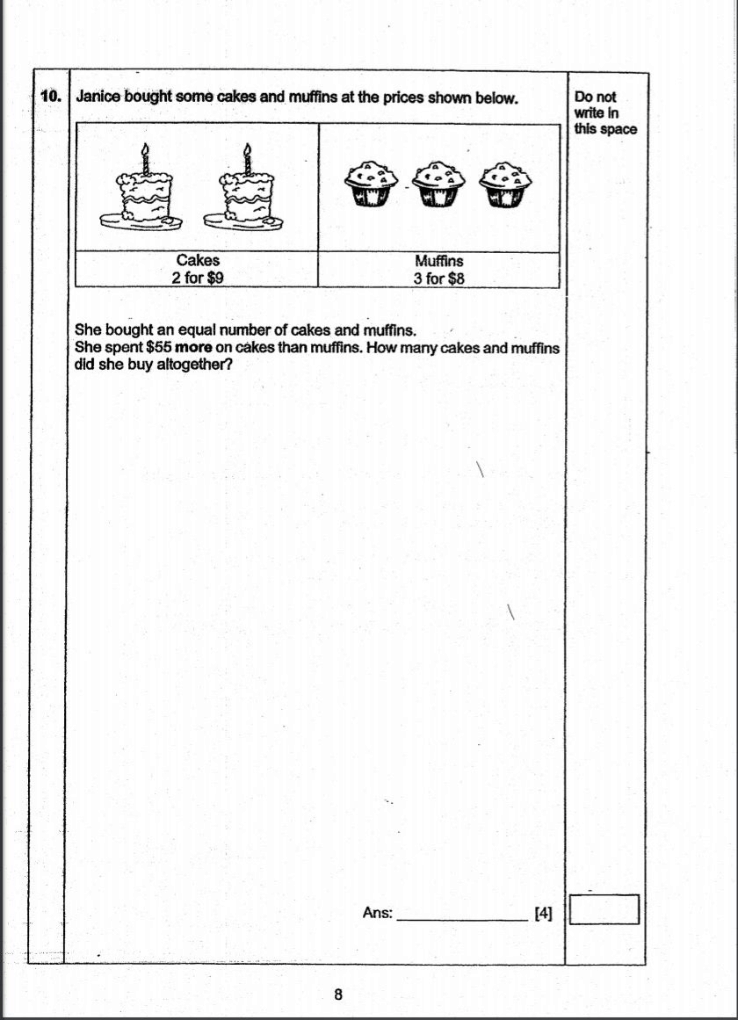# QuestionRelated Link

1 Answer

# Answer

She buys cakes in groups of 2 and muffins in groups of 3. Therefore, in order to have the same amount of cakes and muffins in the end, the ratio of the number of groups of cakes to the number of groups of muffins she bought must be 3 : 2.

 No. of groups Cost per group Total cost Group of 2 cakes 3 units 9 27 units Group of 3 muffins 2 units 8 16 units

Since we know she spent \$55 more on cakes than muffins,

27 units – 16 units = 55
11 units = 55
1 unit = 5

3 units = 15 (No. of groups of 2 cakes)
Total no. of cakes = 15 × 2
= 30

Since no. of cakes and muffins bought is the same,

Total no. of cakes and muffins = 30 × 2
= 60

1 Reply 1 Like

Dear Adwin

Many thanks for your prompt answer.

May i clarify

the ratio of the number of groups of cakes to the number of groups of muffins she bought must be 3 : 2

So that 2 cakes x 3 units = 6

3 muffins x 2 units =6

in order to derive the same number of units purchase?

1 Reply 0 Likes

Adwin, Many thanks.  Sincerely appreciate.

0 Replies 0 Likes